The absence of a time derivative from an equation written in steady-state form reduces the diagonal dominance, and hence convergence, of the resulting matrix equation, as discussed in Sec. 5.5 . Under-relaxation is therefore applied to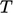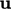, and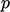to promote convergence in the algorithm in Sec. 5.12 .

Theandﬁelds use equation under-relaxation with factors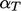and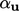, respectively. A value of 0.7 is commonly applied, decreasing to 0.5 for less convergent cases (and sometimes to 0.3 in compressible ﬂow cases, beyond the scope of this book).

Under-relaxation ofis more subtle. The ﬂux corrector requires that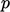is not under-relaxed to ensure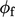obeys mass conservation better. For the momentum corrector, ﬁeld under-relaxation is subsequently applied towith a factor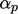. To ﬁnd an optimalfor the momentum corrector, we examine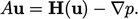(5.22)
This explicit momentum equation contains diagonal coeﬃcients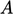and an oﬀ-diagonal contribution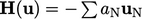from neighbour cell coeﬃcients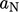and associated velocities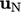.

Convergence is compromised by the explicit nature of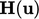. It can be more implicit by “adding and subtracting”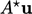, where coeﬃcients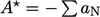are applied to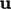in the “owner” cells: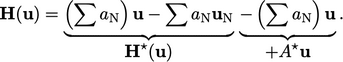(5.23)
Combining Eq. (5.22 ) and Eq. (5.23) gives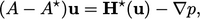(5.24)
which attempts to make Eq. (5.22 ) more implicit in.4 The pressure equation derived from Eq. (5.24) is: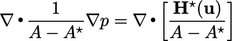(5.25)
This corresponds to under-relaxation of Eq. (5.19 ) by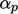, where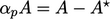. A momentum matrix with coeﬃcients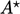is approximately diagonally equal since there is no time derivative. Since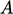represents the diagonal coeﬃcients under-relaxed by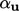,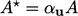. Relating the expressions for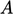and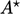gives the optimal under-relaxation factor for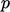for convergence as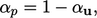(5.26)
leading to the popular choice of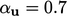and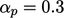.

### Residual control

The algorithm in Sec. 5.12 uses a ﬁxed number of solution steps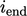. In practice,must be chosen to be large enough to reach an acceptable level of convergence. Once convergence is reached, the simulation should stop to avoid unnecessary computing cost.

A common stopping criterion applies a residual level for each equation, below which the equation is deemed to be converged. When all equations satisfy their respective residual controls, the simulation then stops.

Convergence can also be determined by monitoring any suitable metric, including objective measurements from the simulation, e.g. a force coeﬃcient. When the metric no longer changes signiﬁcantly over subsequent steps, the simulation is stopped.

4Jeﬀ Van Doormaal and George Raithby, Enhancements of the SIMPLE method for predicting incompressible ﬂuid ﬂows, 1984.

Notes on CFD: General Principles - 5.13 Steady-state convergence